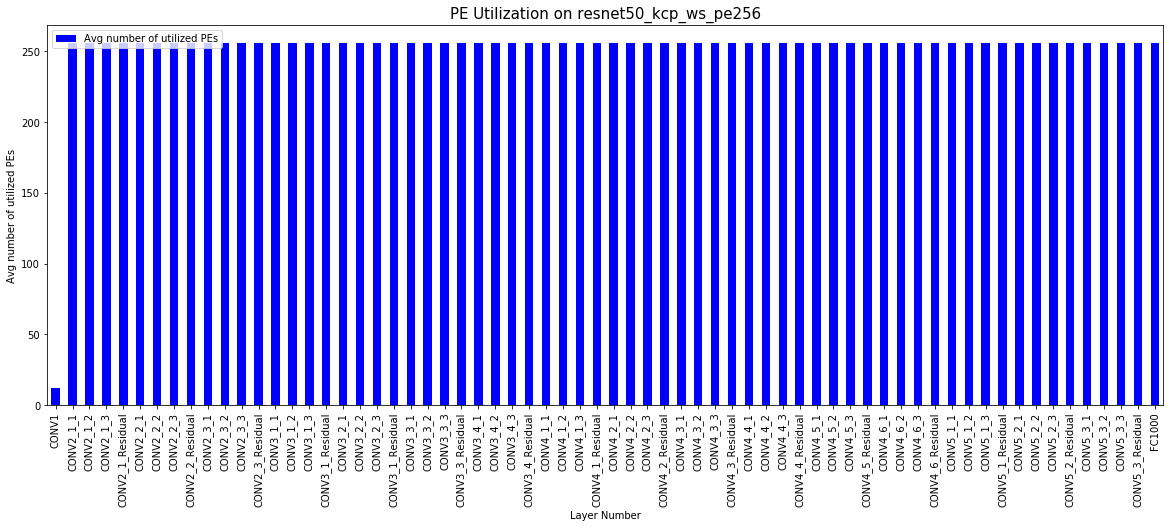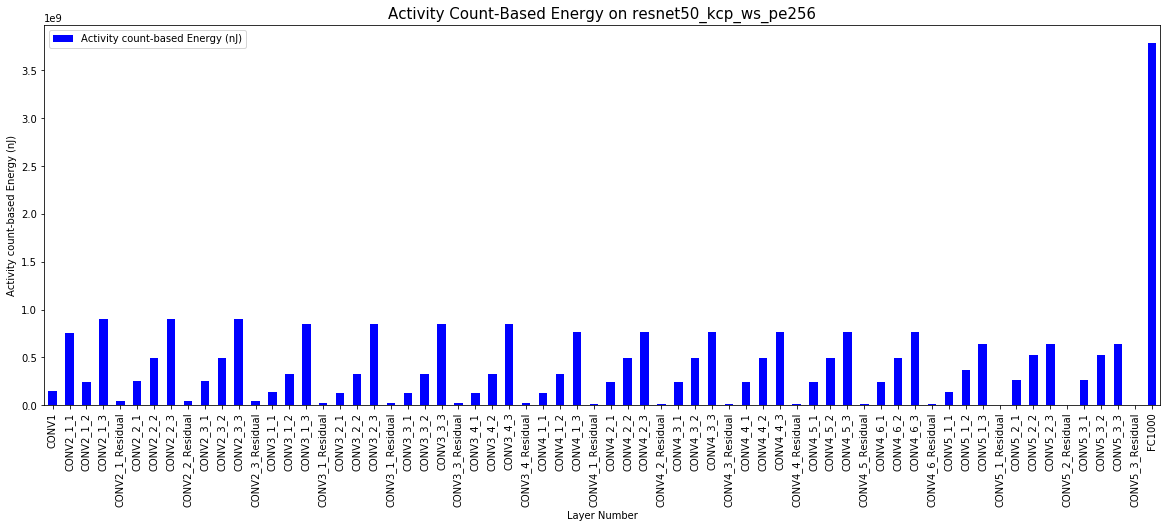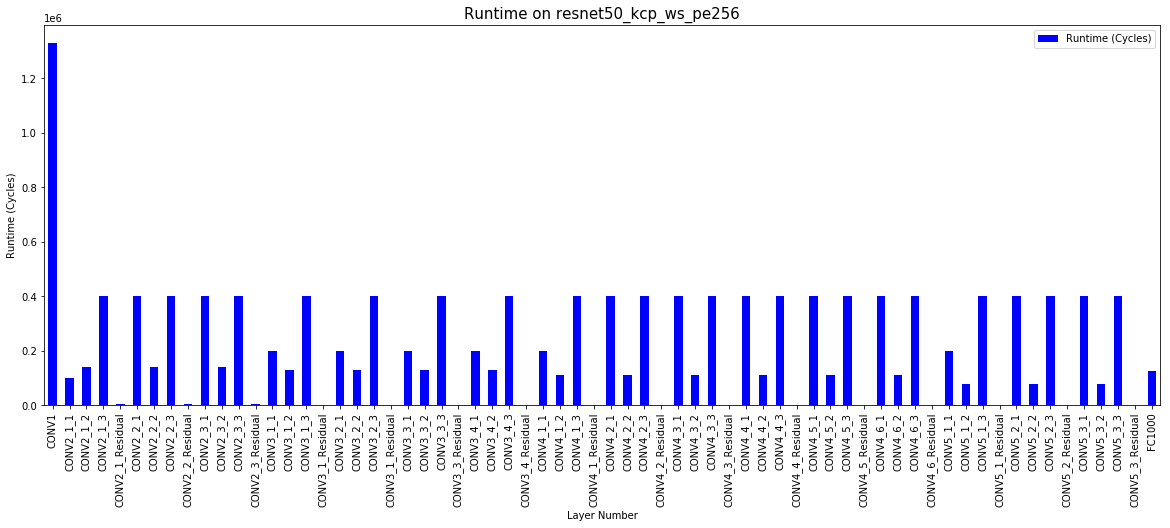# Result Analysis with Jupyter Notebooks¶

Note

This page was generated from maestro_output_analysis.ipynb. To interact with this Jupyter Notebook simply launch from here:## MAESTRO¶

• An Open-source Infrastructure for Modeling Dataflows within Deep Learning Accelerators (https://github.com/georgia-tech-synergy-lab/maestro)

• The throughput and energy efficiency of a dataflow changes dramatically depending on both the DNN topology (i.e., layer shapes and sizes), and accelerator hardware resources (buffer size, and network-on-chip (NoC) bandwidth). This demonstrates the importance of dataflow as a first-order consideration for deep learning accelerator ASICs, both at design-time when hardware resources (buffers and interconnects) are being allocated on-chip, and compile-time when different layers need to be optimally mapped for high utilization and energy-efficiency.

## MAESTRO Result Analysis¶

• This script is written to analyse the detailed output of Maestro per layer using various graphing techniques to showcase interesting results and filter out important results from the gigantic output file that the tool dumps emphasising the importance of Hardware Requirements and Dataflow used per layer for a DNN Model.

### 1 - Packages¶

Let’s first import all the packages that you will need during this assignment. - numpy is the fundamental package for scientific computing with Python. - matplotlib is a library to plot graphs in Python. - pandas is a fast, powerful and easy to use open source data analysis and manipulation tool

```%matplotlib inline
import numpy as np
import pandas as pd
import matplotlib.pyplot as plt
import import_ipynb
from Util_graph import draw_graph
```
```importing Jupyter notebook from Util_graph.ipynb
```

### 2 - Reading the output file¶

Please provide the output file for your Maestro run to be read by the script with the path relative to the script.

As an example we are reading Resnet50_dla.csv

```new_data = pd.read_csv('Resnet50_dla.csv')
```

### 2 - Creating Graphs¶

Graphs can be generated by assigning values to the variables provided below in the code.

The graphs are created by draw_graph function which operates on the variables provided below.

### 3- Understanding the Variables¶

• x = “x axis of your graph”

• One can simply assign the column name from the csv to this graph as shown below in the code. Also, an alternate way is to select the column number from dataframe.columns. For example: for this case it will be new_data.columns that corresponds to ‘Layer Number’.

• y = “y axis of your graph”

• One can simply assign the column name from the csv to this graph as shown below in the code. Also, an alternate way is to select the column number from dataframe.columns. For example: for this case it will be new_data.columns that corresponds to ‘Throughput (MACs/Cycle)’.

• User can compare multiple bar graphs by providing a list to y.

• color = “color of the graph”

• figsize = “Controls the size of the graph”

• Legend =” Do you want a legend or not” -> values (True/False)

• title = “title of your graph”

• xlabel = “xlabel name on the graph”

• ylabel = “ylabel name on the graph”

• start_layer = “start layer that you want to analyse”

• a subset of layers to be plotted which are of interest. This variable allows you to do that.

• end_layer =”last layer that you want to analyse”

• a subset of layers to be plotted which are of interest. This variable allows you to do that. Please provide “all” if you want all the layers to be seen in the graph

```x = 'Layer Number'
y = 'Throughput (MACs/Cycle)'
color = 'blue'
figsize = (20,10)
legend = 'true'
title = 'Throughput vs Layer'
xlabel = x
ylabel = y
start_layer = 0
end_layer = 'all'
draw_graph(new_data, y, x, color, figsize, legend, title, xlabel, ylabel, start_layer, end_layer)
```
```<matplotlib.axes._subplots.AxesSubplot at 0x152d950c828>
``````x = 'Layer Number'
y = 'Activity count-based Energy (nJ)'
color = 'red'
figsize = (20,10)
legend = 'true'
title = 'Energy vs Layer'
xlabel = x
ylabel = y
start_layer = 0
end_layer = 'all'
draw_graph(new_data, y, x, color, figsize, legend, title, xlabel, ylabel, start_layer, end_layer)
```
```<matplotlib.axes._subplots.AxesSubplot at 0x152d98bfdd8>
``````x = 'Layer Number'
y = 'Throughput Per Energy (GMACs/s*J)'
color = 'green'
figsize = (20,10)
legend = 'true'
title = 'Throughput per Energy vs Layer'
xlabel = x
ylabel = y
start_layer = 0
end_layer = 'all'
draw_graph(new_data, y, x, color, figsize, legend, title, xlabel, ylabel, start_layer, end_layer)
```
```<matplotlib.axes._subplots.AxesSubplot at 0x152d99a49b0>
``````x = 'Layer Number'
y = ['Throughput (MACs/Cycle)', 'Throughput (MACs/Cycle)']
color = ['blue','yellow']
figsize = (20,10)
legend = 'true'
title = 'Throughput vs Layer'
xlabel = x
ylabel = 'Throughput (MACs/Cycle)'
start_layer = 0
end_layer = 10
draw_graph(new_data, y, x, color, figsize, legend, title, xlabel, ylabel, start_layer, end_layer)
```
```<matplotlib.axes._subplots.AxesSubplot at 0x152d66e7c50>
```